# R S Aggarwal Solutions for Class 11 Maths Chapter 21 Circles

R S Aggarwal Class 11 Maths Solutions for Chapter 11 are provided here. In this chapter, students will learn how to find the equation of a circle under different conditions.

Important Points to Remember:

1. The general form of the equation of a circle : (x – h)2 + (y – k)2 = r2

Where, r is the radius of the circle and (h, k) is the centre of the circle.

2. The equation of a circle with centre at the origin and radius r is given by

x2 + y2 = r2

3. The equation of a circle with A(x1 , y1 ) and B(x2 , y2) as the end points of a diameter is given by (x – x1)(x – x2) + (y – y1)(y – y2) = 0

Check the detailed R S Aggarwal Solutions for all Class 11 chapters and start practicing to score good marks.

## Download PDF of R S Aggarwal Solutions for Class 11 Maths Chapter 21 Circles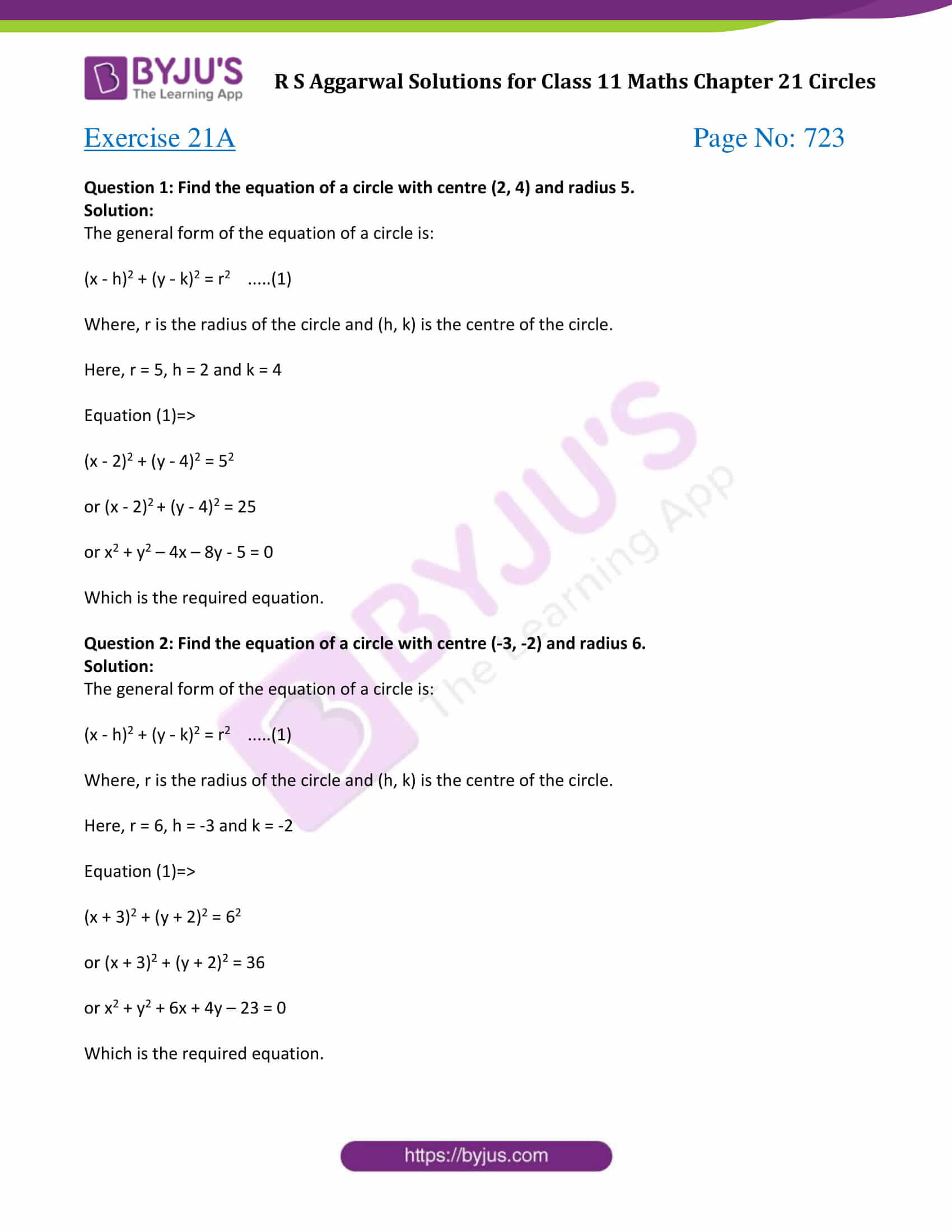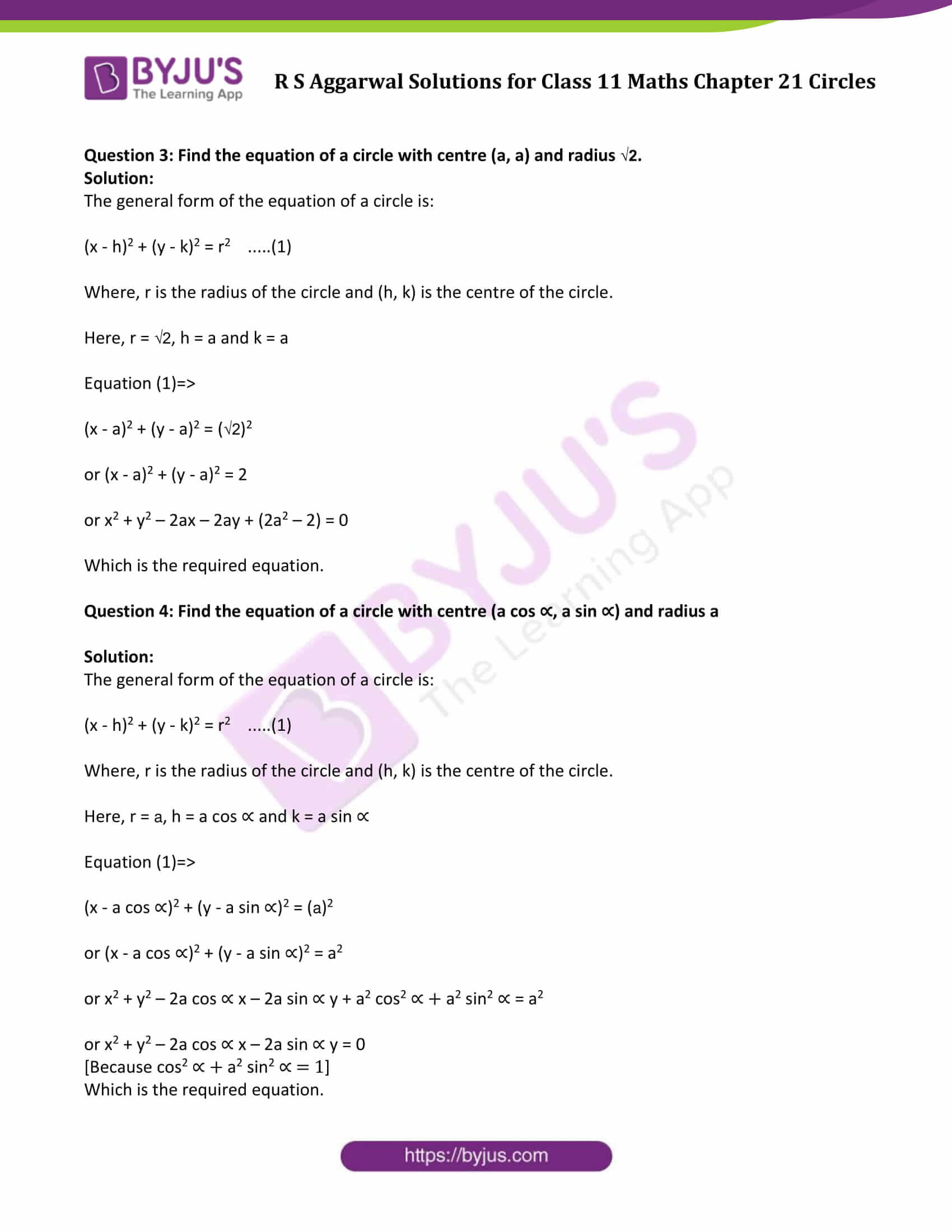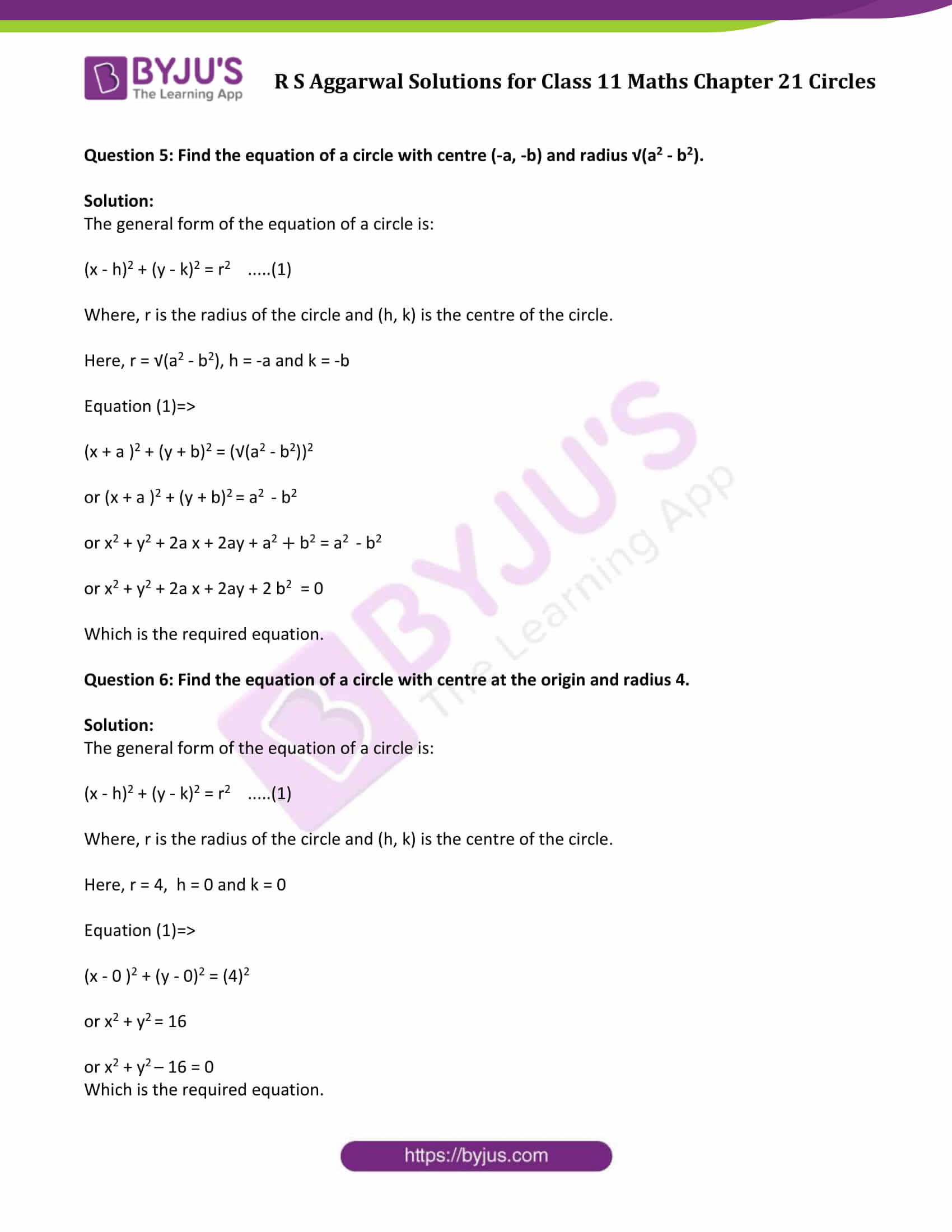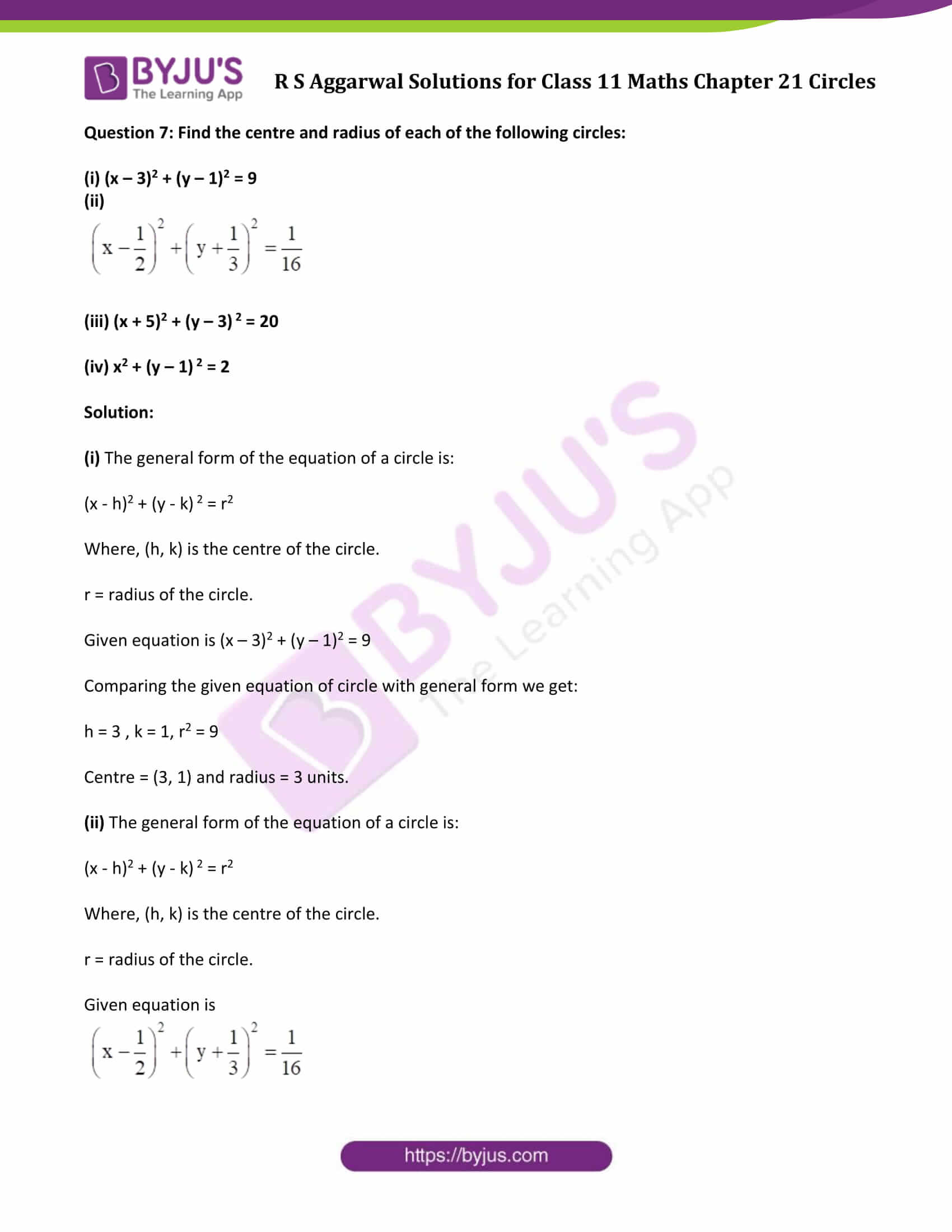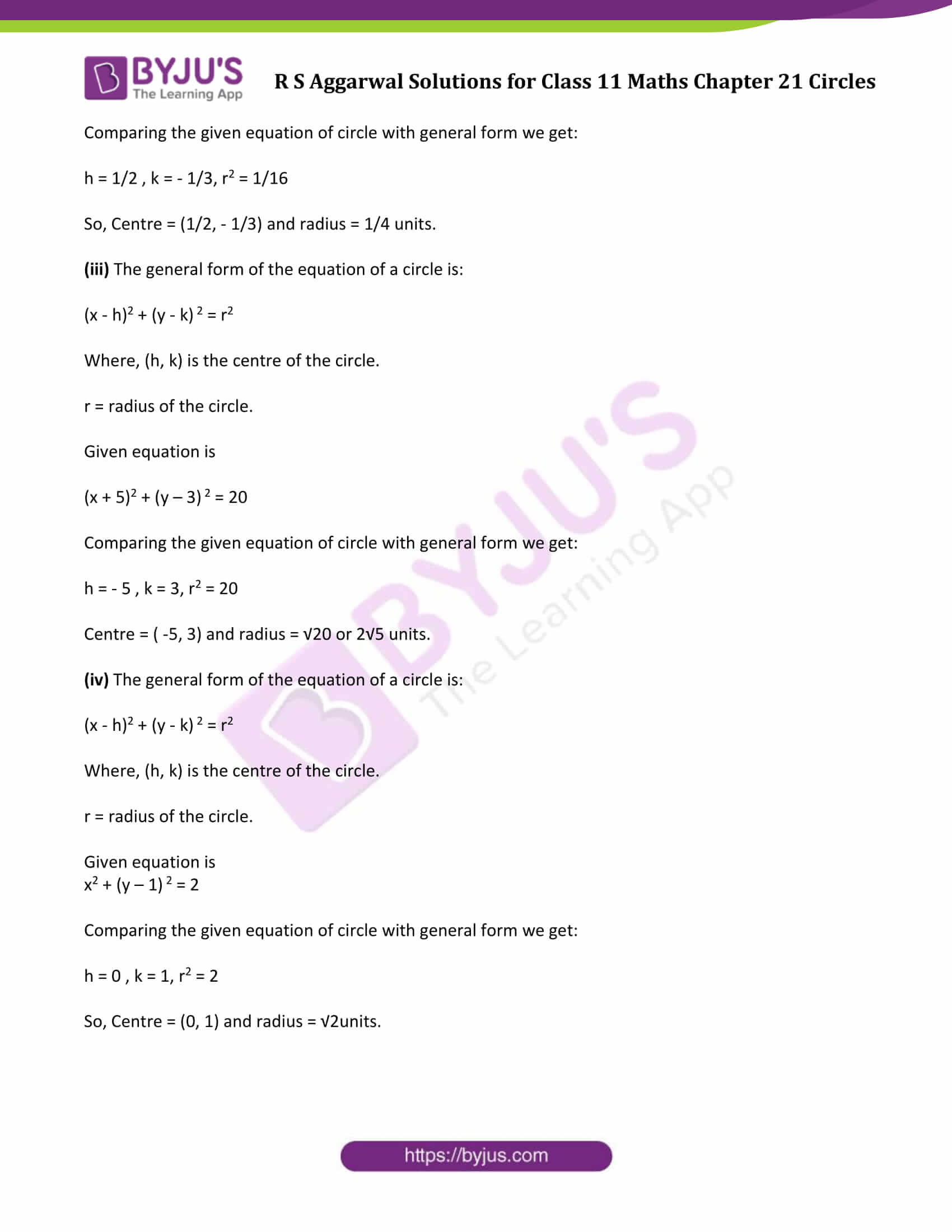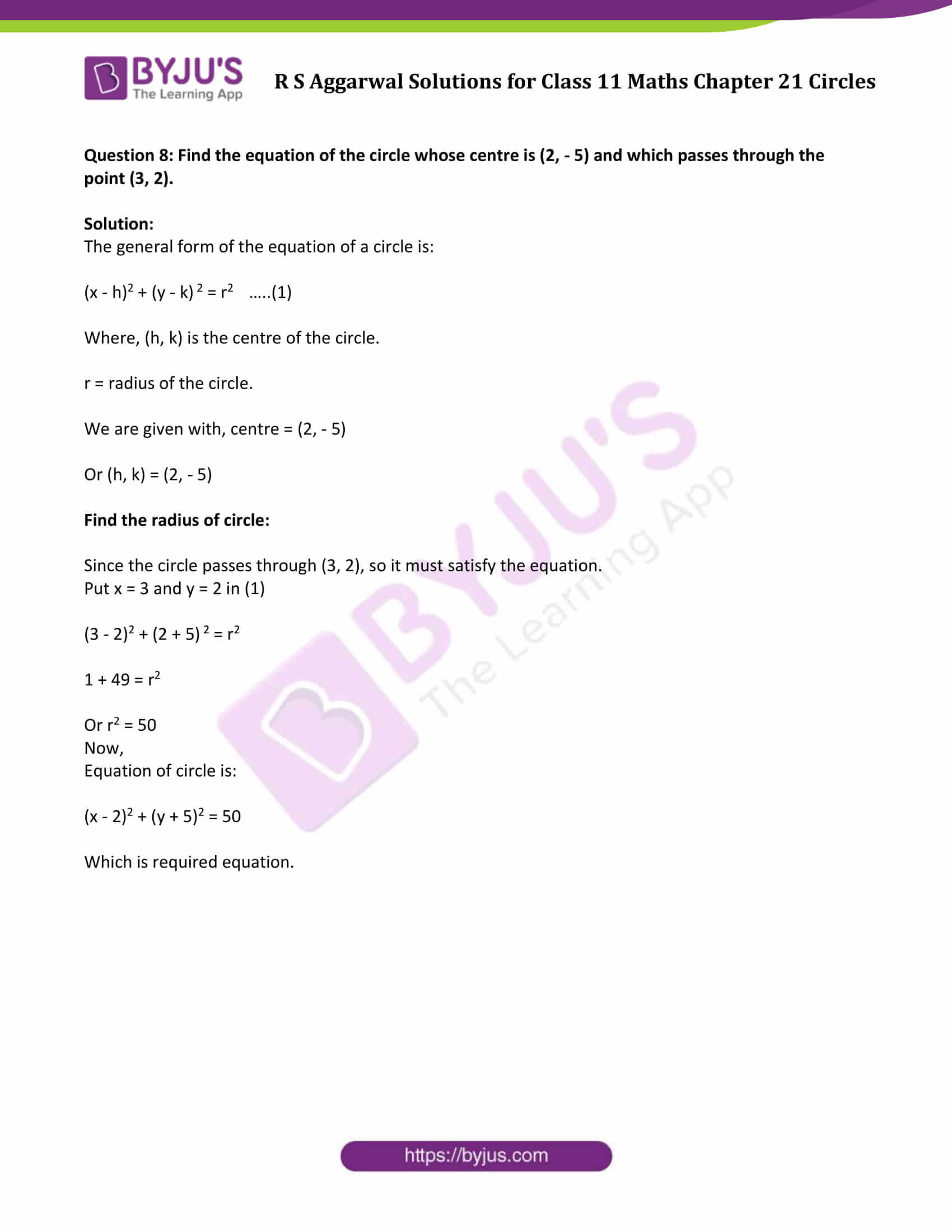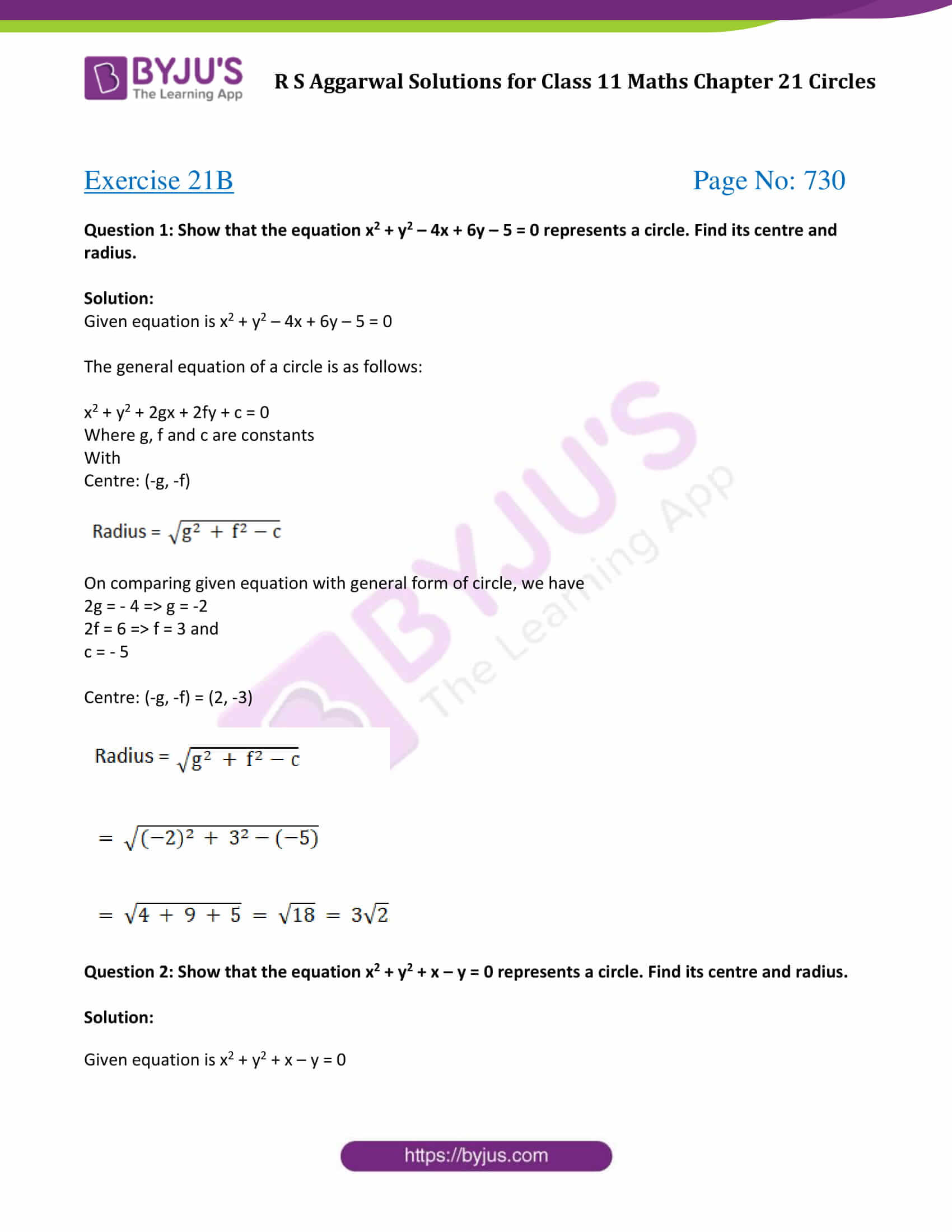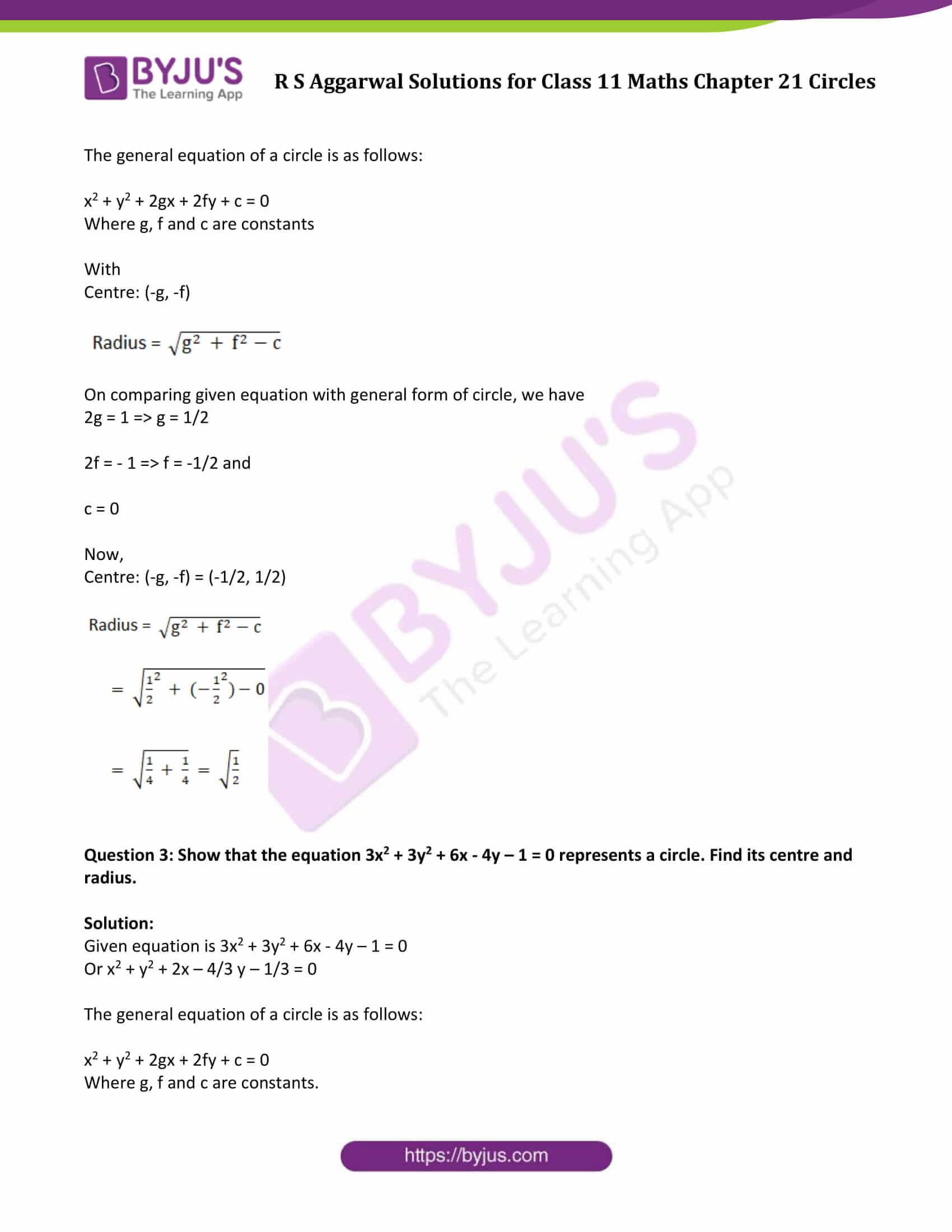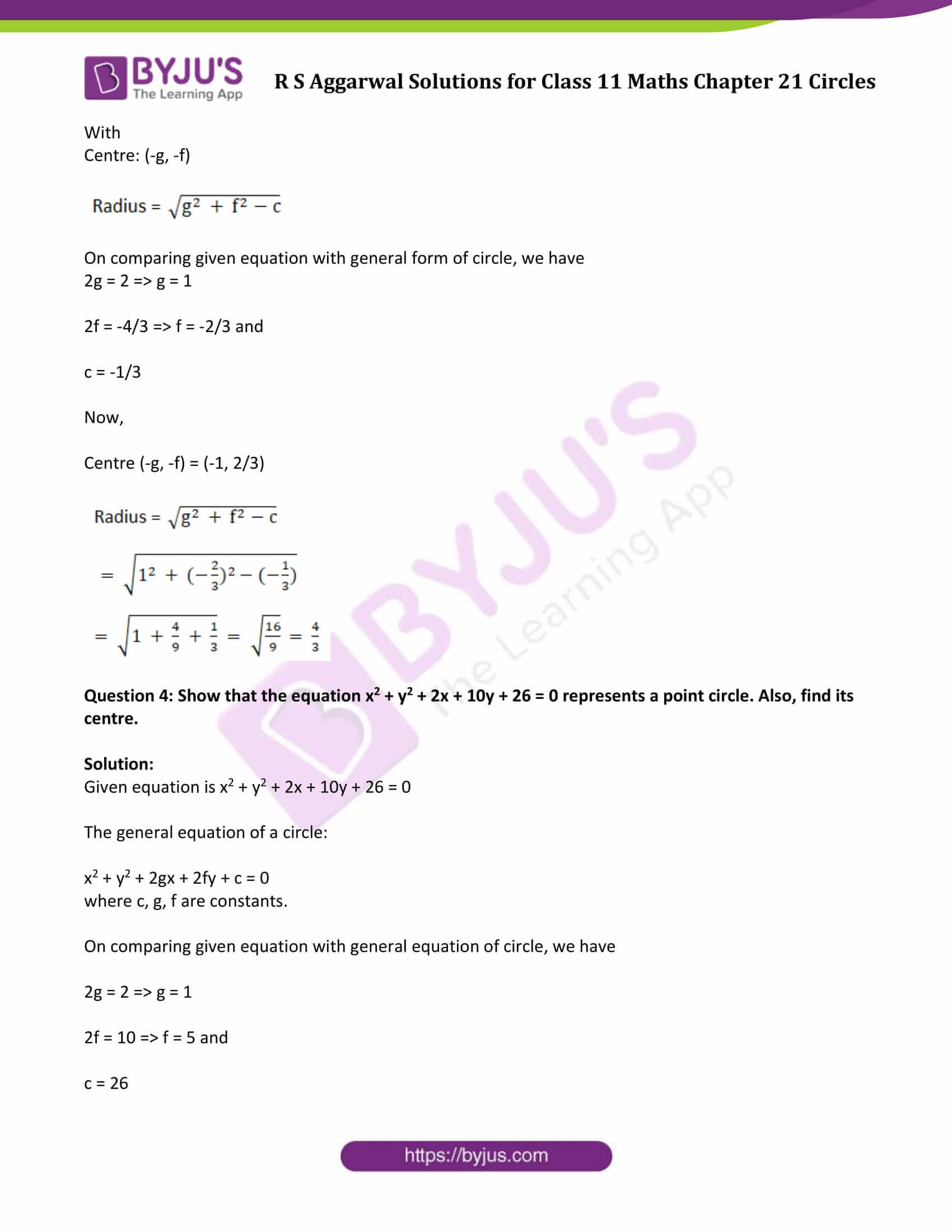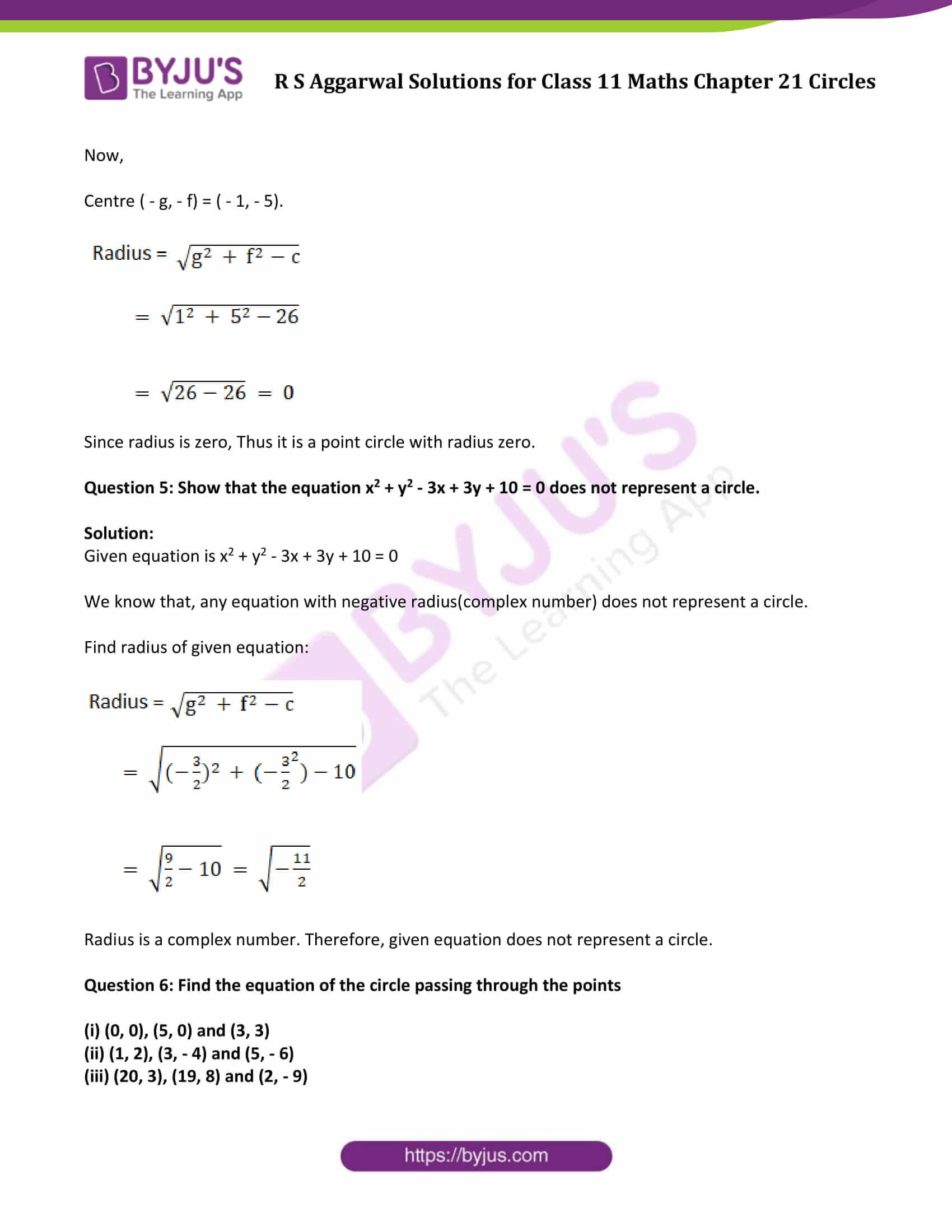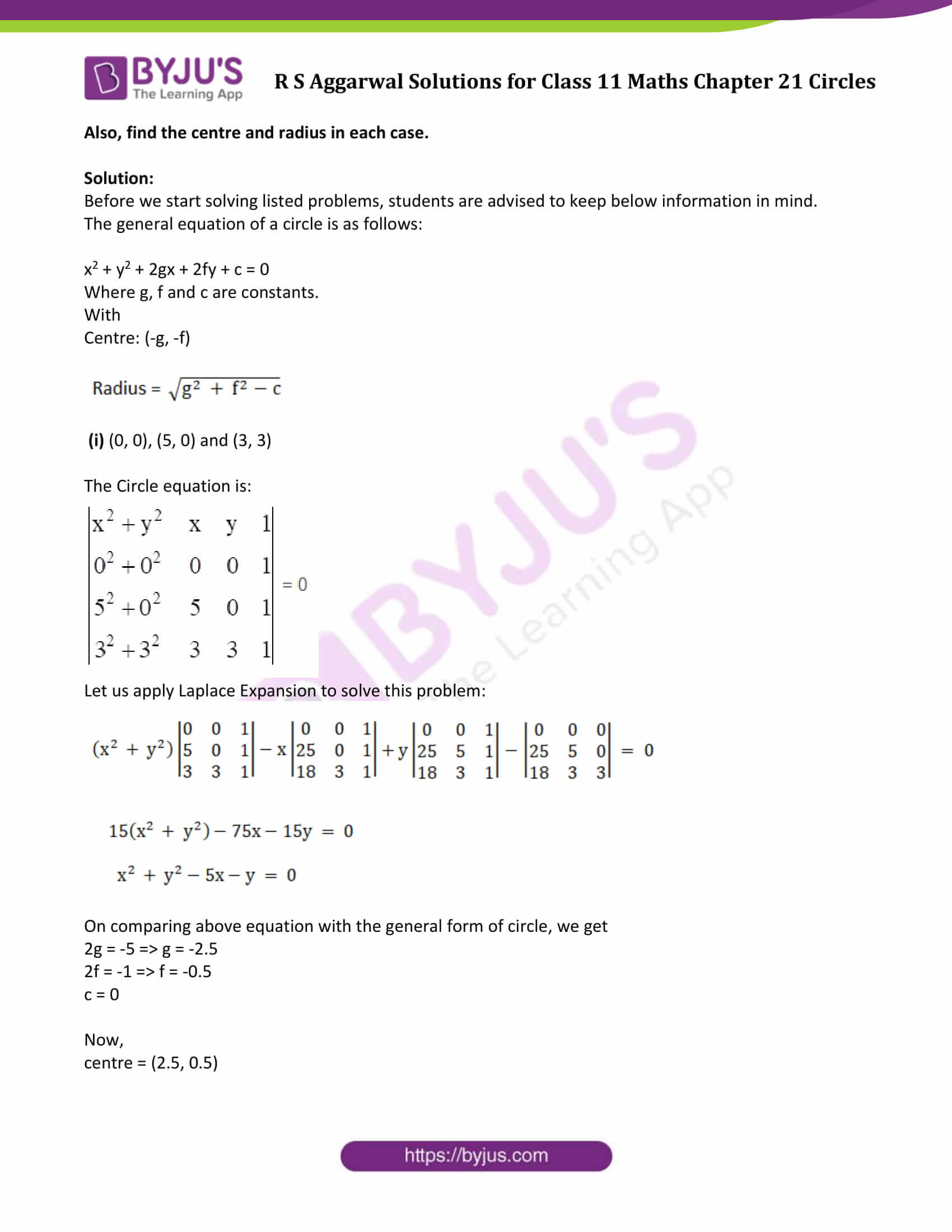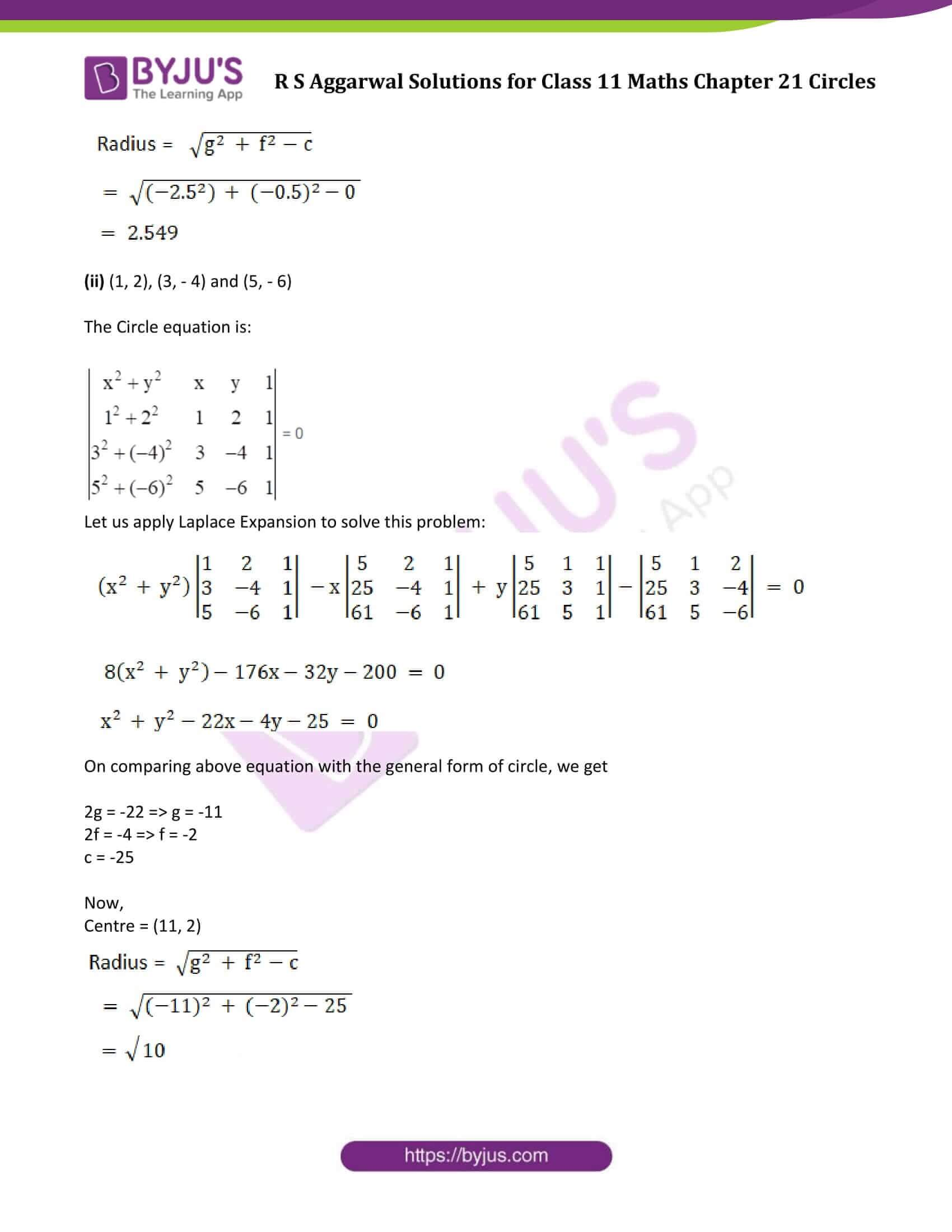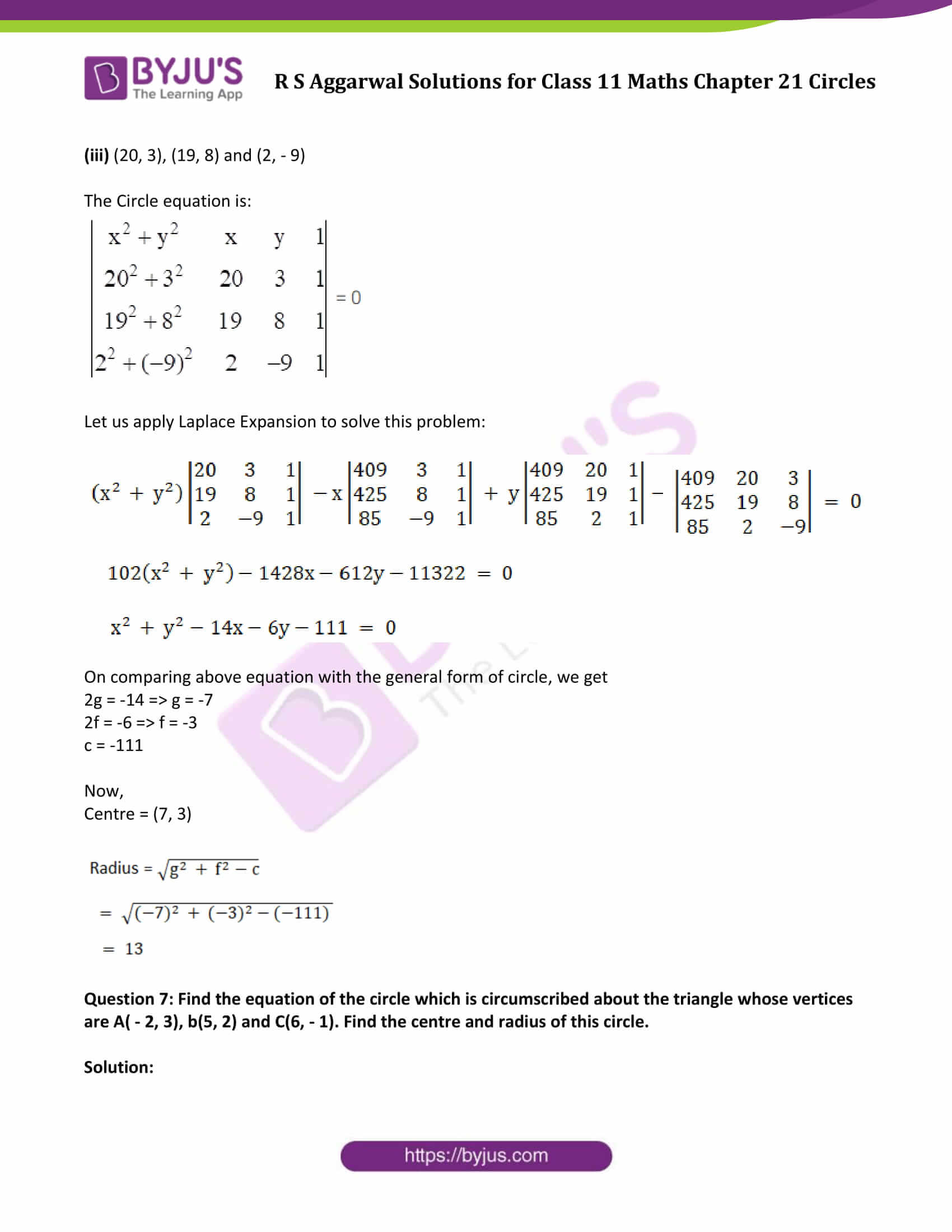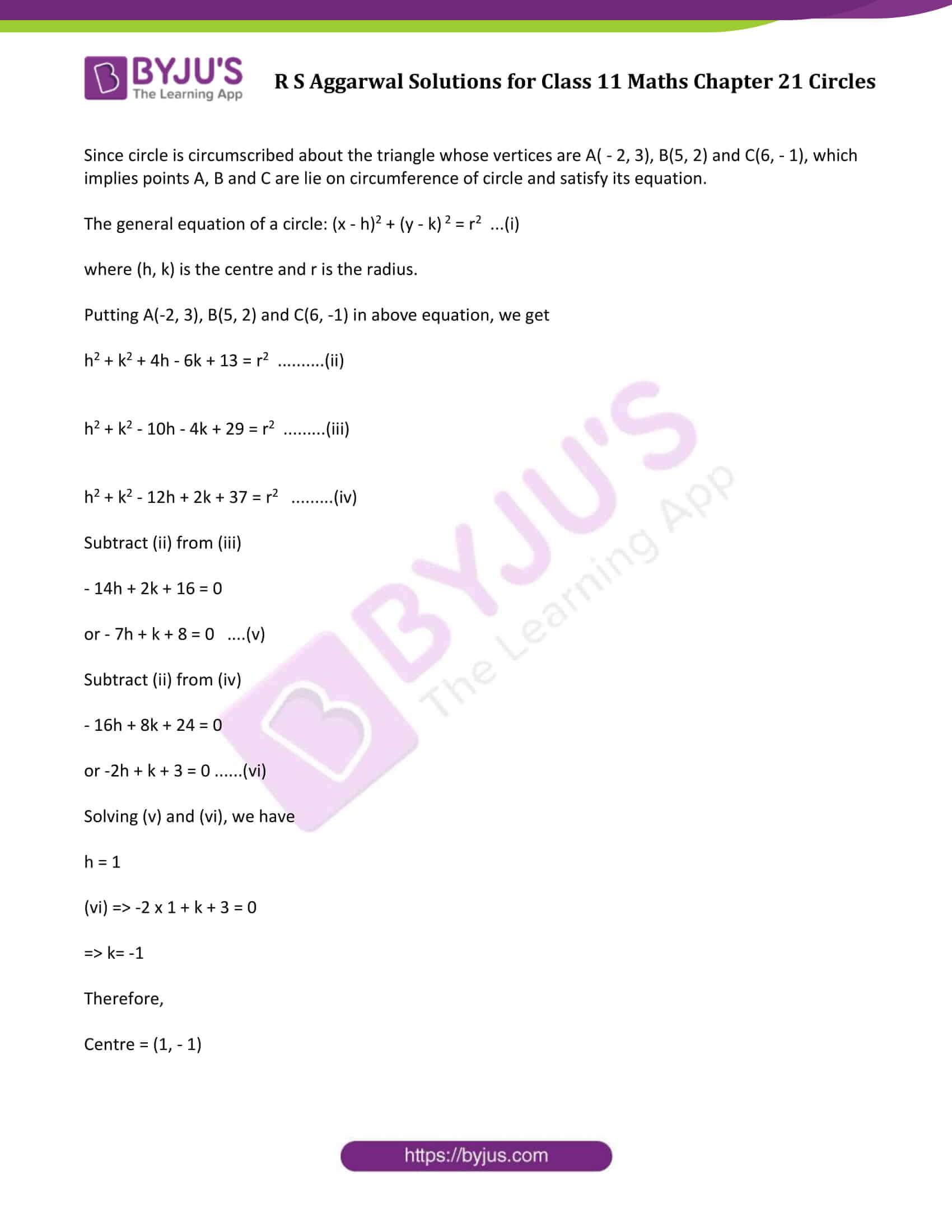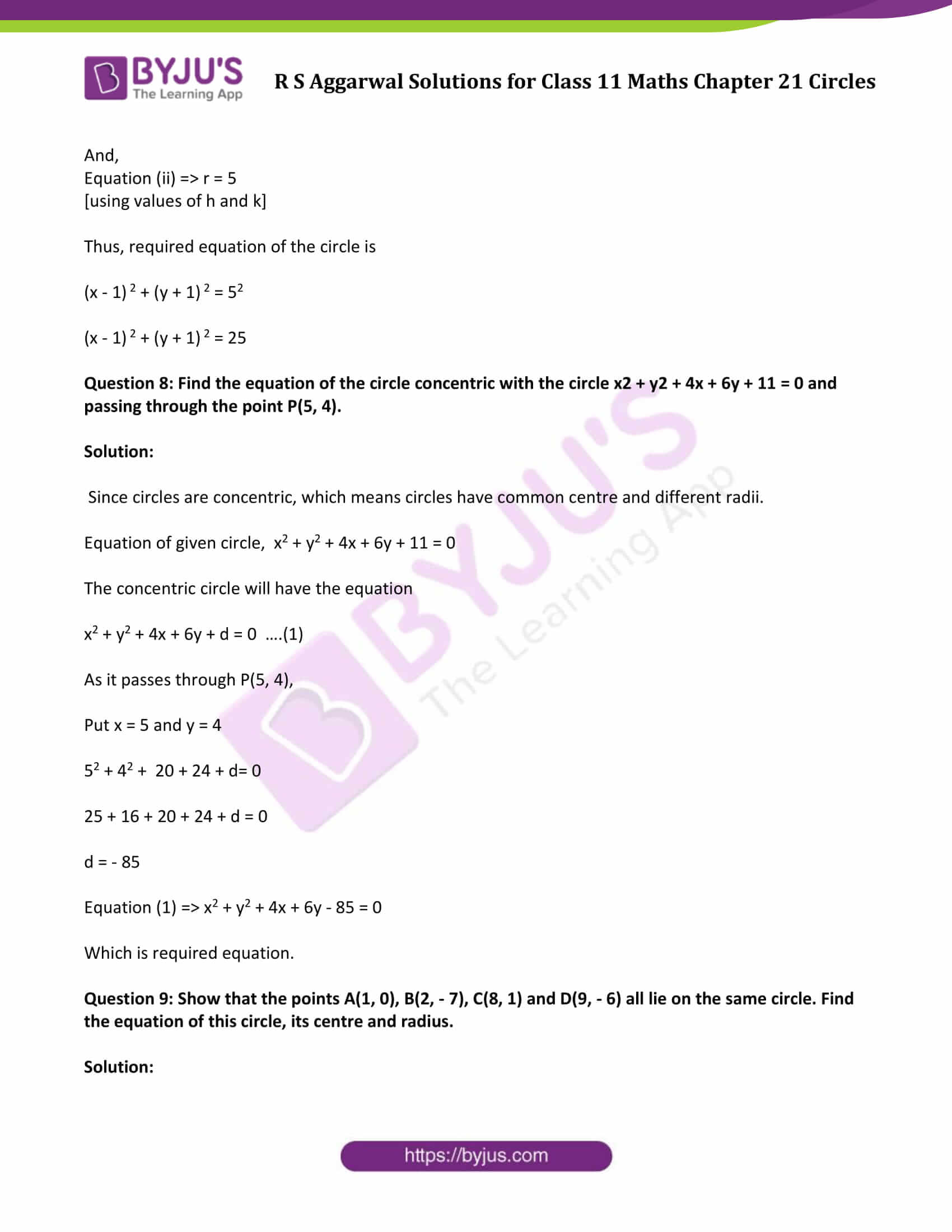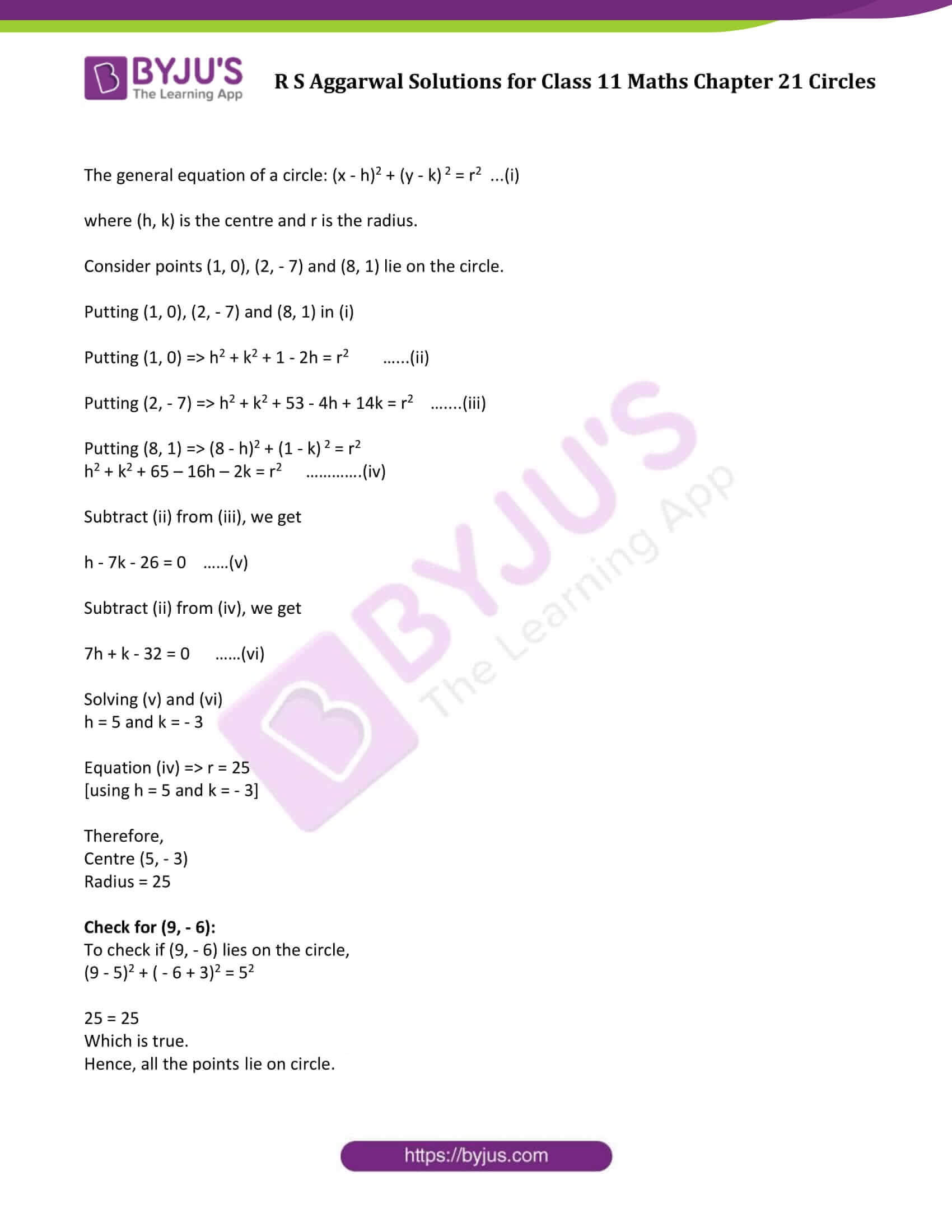### Access Answers of R S Aggarwal Solutions for Class 11 Maths Chapter 21 Circles

Exercise 21A Page No: 723

Question 1: Find the equation of a circle with centre (2, 4) and radius 5.

Solution:

The general form of the equation of a circle is:

(x – h)2 + (y – k)2 = r2 …..(1)

Where, r is the radius of the circle and (h, k) is the centre of the circle.

Here, r = 5, h = 2 and k = 4

Equation (1)⇒

(x – 2)2 + (y – 4)2 = 52

or (x – 2)2 + (y – 4)2 = 25

or x2 + y2 – 4x – 8y – 5 = 0

Which is the required equation.

Question 2: Find the equation of a circle with centre (-3, -2) and radius 6.

Solution:

The general form of the equation of a circle is:

(x – h)2 + (y – k)2 = r2 …..(1)

Where, r is the radius of the circle and (h, k) is the centre of the circle.

Here, r = 6, h = -3 and k = -2

Equation (1)⇒

(x + 3)2 + (y + 2)2 = 62

or (x + 3)2 + (y + 2)2 = 36

or x2 + y2 + 6x + 4y – 23 = 0

Which is the required equation.

Question 3: Find the equation of a circle with centre (a, a) and radius √2.

Solution:

The general form of the equation of a circle is:

(x – h)2 + (y – k)2 = r2 …..(1)

Where, r is the radius of the circle and (h, k) is the centre of the circle.

Here, r = √2, h = a and k = a

Equation (1)⇒

(x – a)2 + (y – a)2 = (√2)2

or (x – a)2 + (y – a)2 = 2

or x2 + y2 – 2ax – 2ay + (2a2 – 2) = 0

Which is the required equation.

Question 4: Find the equation of a circle with centre (a cos ∝, a sin ∝) and radius a

Solution:

The general form of the equation of a circle is:

(x – h)2 + (y – k)2 = r2 …..(1)

Where, r is the radius of the circle and (h, k) is the centre of the circle.

Here, r = a, h = a cos ∝ and k = a sin ∝

Equation (1)⇒

(x – a cos ∝)2 + (y – a sin ∝)2 = (a)2

or (x – a cos ∝)2 + (y – a sin ∝)2 = a2

or x2 + y2 – 2a cos ∝ x – 2a sin ∝ y + a2 cos2 ∝ + a2 sin2 ∝ = a2

or x2 + y2 – 2a cos ∝ x – 2a sin ∝ y = 0

[Because cos2 ∝ + a2 sin2 ∝ = 1]

Which is the required equation.

Question 5: Find the equation of a circle with centre (-a, -b) and radius √(a2 – b2).

Solution:

The general form of the equation of a circle is:

(x – h)2 + (y – k)2 = r2 …..(1)

Where, r is the radius of the circle and (h, k) is the centre of the circle.

Here, r = √(a2 – b2), h = -a and k = -b

Equation (1)⇒

(x + a )2 + (y + b)2 = (√(a2 – b2))2

or (x + a )2 + (y + b)2 = a2 – b2

or x2 + y2 + 2a x + 2ay + a2 + b2 = a2 – b2

or x2 + y2 + 2a x + 2ay + 2 b2 = 0

Which is the required equation.

Question 6: Find the equation of a circle with centre at the origin and radius 4.

Solution:

The general form of the equation of a circle is:

(x – h)2 + (y – k)2 = r2 …..(1)

Where, r is the radius of the circle and (h, k) is the centre of the circle.

Here, r = 4, h = 0 and k = 0

Equation (1)⇒

(x – 0 )2 + (y – 0)2 = (4)2

or x2 + y2 = 16

or x2 + y2 – 16 = 0

Which is the required equation.

Question 7: Find the centre and radius of each of the following circles:

(i) (x – 3)2 + (y – 1)2 = 9

(ii)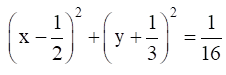(iii) (x + 5)2 + (y – 3) 2 = 20

(iv) x2 + (y – 1) 2 = 2

Solution:

(i) The general form of the equation of a circle is:

(x – h)2 + (y – k) 2 = r2

Where, (h, k) is the centre of the circle.

r = radius of the circle.

Given equation is (x – 3)2 + (y – 1)2 = 9

Comparing the given equation of circle with general form we get:

h = 3 , k = 1, r2 = 9

Centre = (3, 1) and radius = 3 units.

(ii) The general form of the equation of a circle is:

(x – h)2 + (y – k) 2 = r2

Where, (h, k) is the centre of the circle.

r = radius of the circle.

Given equation isComparing the given equation of circle with general form we get:

h = 1/2 , k = – 1/3, r2 = 1/16

So, Centre = (1/2, – 1/3) and radius = 1/4 units.

(iii) The general form of the equation of a circle is:

(x – h)2 + (y – k) 2 = r2

Where, (h, k) is the centre of the circle.

r = radius of the circle.

Given equation is

(x + 5)2 + (y – 3) 2 = 20

Comparing the given equation of circle with general form we get:

h = – 5 , k = 3, r2 = 20

Centre = ( -5, 3) and radius = √20 or 2√5 units.

(iv) The general form of the equation of a circle is:

(x – h)2 + (y – k) 2 = r2

Where, (h, k) is the centre of the circle.

r = radius of the circle.

Given equation is

x2 + (y – 1) 2 = 2

Comparing the given equation of circle with general form we get:

h = 0 , k = 1, r2 = 2

So, Centre = (0, 1) and radius = √2units.

Question 8: Find the equation of the circle whose centre is (2, – 5) and which passes through the point (3, 2).

Solution:

The general form of the equation of a circle is:

(x – h)2 + (y – k) 2 = r2 …..(1)

Where, (h, k) is the centre of the circle.

r = radius of the circle.

We are given with, centre = (2, – 5)

Or (h, k) = (2, – 5)

Since the circle passes through (3, 2), so it must satisfy the equation.

Put x = 3 and y = 2 in (1)

(3 – 2)2 + (2 + 5) 2 = r2

1 + 49 = r2

Or r2 = 50

Now,

Equation of circle is:

(x – 2)2 + (y + 5)2 = 50

Which is required equation.

Exercise 21B Page No: 730

Question 1: Show that the equation x2 + y2 – 4x + 6y – 5 = 0 represents a circle. Find its centre and radius.

Solution:

Given equation is x2 + y2 – 4x + 6y – 5 = 0

The general equation of a circle is as follows:

x2 + y2 + 2gx + 2fy + c = 0

Where g, f and c are constants

With

Centre: (-g, -f)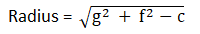On comparing given equation with general form of circle, we have

2g = – 4 ⇒ g = -2

2f = 6 ⇒ f = 3 and

c = – 5

Centre: (-g, -f) = (2, -3)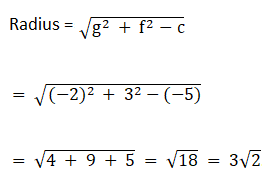Question 2: Show that the equation x2 + y2 + x – y = 0 represents a circle. Find its centre and radius.

Solution:

Given equation is x2 + y2 + x – y = 0

The general equation of a circle is as follows:

x2 + y2 + 2gx + 2fy + c = 0

Where g, f and c are constants

With

Centre: (-g, -f)On comparing given equation with general form of circle, we have

2g = 1 ⇒ g = 1/2

2f = – 1 ⇒ f = -1/2 and

c = 0

Now,

Centre: (-g, -f) = (-1/2, 1/2)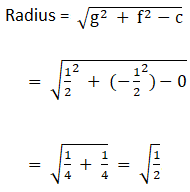Question 3: Show that the equation 3x2 + 3y2 + 6x – 4y – 1 = 0 represents a circle. Find its centre and radius.

Solution:

Given equation is 3x2 + 3y2 + 6x – 4y – 1 = 0

Or x2 + y2 + 2x – 4/3 y – 1/3 = 0

The general equation of a circle is as follows:

x2 + y2 + 2gx + 2fy + c = 0

Where g, f and c are constants.

With

Centre: (-g, -f)On comparing given equation with general form of circle, we have

2g = 2 ⇒ g = 1

2f = -4/3 ⇒ f = -2/3 and

c = -1/3

Now,

Centre (-g, -f) = (-1, 2/3)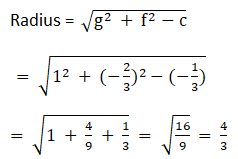Question 4: Show that the equation x2 + y2 + 2x + 10y + 26 = 0 represents a point circle. Also, find its centre.

Solution:

Given equation is x2 + y2 + 2x + 10y + 26 = 0

The general equation of a circle:

x2 + y2 + 2gx + 2fy + c = 0

where c, g, f are constants.

On comparing given equation with general equation of circle, we have

2g = 2 ⇒ g = 1

2f = 10 ⇒ f = 5 and

c = 26

Now,

Centre ( – g, – f) = ( – 1, – 5).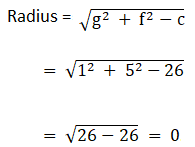Since radius is zero, Thus it is a point circle with radius zero.

Question 5: Show that the equation x2 + y2 – 3x + 3y + 10 = 0 does not represent a circle.

Solution:

Given equation is x2 + y2 – 3x + 3y + 10 = 0

We know that, any equation with negative radius(complex number) does not represent a circle.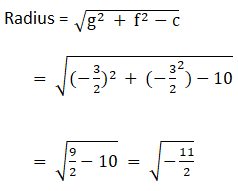Radius is a complex number. Therefore, given equation does not represent a circle.

Question 6: Find the equation of the circle passing through the points

(i) (0, 0), (5, 0) and (3, 3)

(ii) (1, 2), (3, – 4) and (5, – 6)

(iii) (20, 3), (19, 8) and (2, – 9)

Also, find the centre and radius in each case.

Solution:

Before we start solving listed problems, students are advised to keep below information in mind.

The general equation of a circle is as follows:

x2 + y2 + 2gx + 2fy + c = 0

Where g, f and c are constants.

With

Centre: (-g, -f)(i) (0, 0), (5, 0) and (3, 3)

The Circle equation is: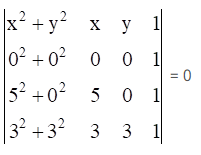Let us apply Laplace Expansion to solve this problem: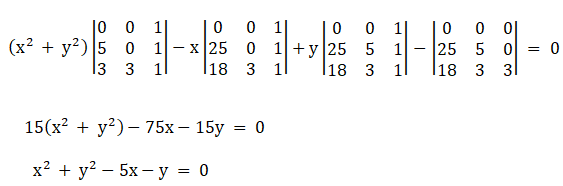On comparing above equation with the general form of circle, we get

2g = -5 ⇒ g = -2.5

2f = -1 ⇒ f = -0.5

c = 0

Now,

centre = (2.5, 0.5)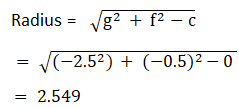(ii) (1, 2), (3, – 4) and (5, – 6)

The Circle equation is: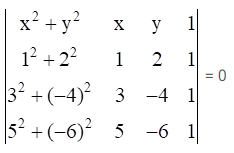Let us apply Laplace Expansion to solve this problem: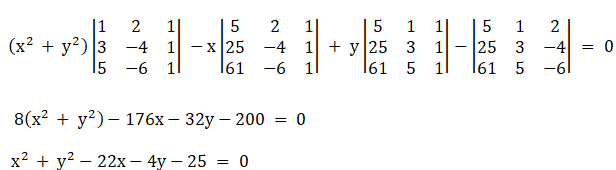On comparing above equation with the general form of circle, we get

2g = -22 ⇒ g = -11

2f = -4 ⇒ f = -2

c = -25

Now,

Centre = (11, 2)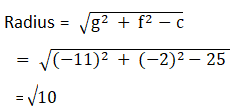(iii) (20, 3), (19, 8) and (2, – 9)

The Circle equation is: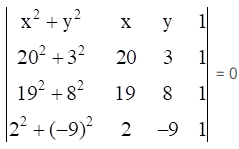Let us apply Laplace Expansion to solve this problem: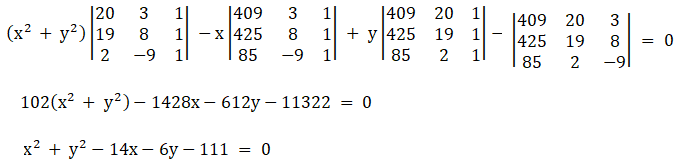On comparing above equation with the general form of circle, we get

2g = -14 ⇒ g = -7

2f = -6 ⇒ f = -3

c = -111

Now,

Centre = (7, 3)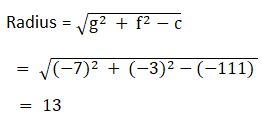Question 7: Find the equation of the circle which is circumscribed about the triangle whose vertices are A( – 2, 3), b(5, 2) and C(6, – 1). Find the centre and radius of this circle.

Solution:

Since circle is circumscribed about the triangle whose vertices are A( – 2, 3), B(5, 2) and C(6, – 1), which implies points A, B and C are lie on circumference of circle and satisfy its equation.

The general equation of a circle: (x – h)2 + (y – k) 2 = r2 …(i)

where (h, k) is the centre and r is the radius.

Putting A(-2, 3), B(5, 2) and C(6, -1) in above equation, we get

h2 + k2 + 4h – 6k + 13 = r2 ……….(ii)

h2 + k2 – 10h – 4k + 29 = r2 ………(iii)

h2 + k2 – 12h + 2k + 37 = r2 ………(iv)

Subtract (ii) from (iii)

– 14h + 2k + 16 = 0

or – 7h + k + 8 = 0 ….(v)

Subtract (ii) from (iv)

– 16h + 8k + 24 = 0

or -2h + k + 3 = 0 ……(vi)

Solving (v) and (vi), we have

h = 1

(vi) ⇒ -2 x 1 + k + 3 = 0

⇒ k= -1

Therefore,

Centre = (1, – 1)

And,

Equation (ii) ⇒ r = 5

[using values of h and k]

Thus, required equation of the circle is

(x – 1) 2 + (y + 1) 2 = 52

(x – 1) 2 + (y + 1) 2 = 25

Question 8: Find the equation of the circle concentric with the circle x2 + y2 + 4x + 6y + 11 = 0 and passing through the point P(5, 4).

Solution:

Since circles are concentric, which means circles have common centre and different radii.

Equation of given circle, x2 + y2 + 4x + 6y + 11 = 0

The concentric circle will have the equation

x2 + y2 + 4x + 6y + d = 0 ….(1)

As it passes through P(5, 4),

Put x = 5 and y = 4

52 + 42 + 20 + 24 + d= 0

25 + 16 + 20 + 24 + d = 0

d = – 85

Equation (1) ⇒ x2 + y2 + 4x + 6y – 85 = 0

Which is required equation.

Question 9: Show that the points A(1, 0), B(2, – 7), C(8, 1) and D(9, – 6) all lie on the same circle. Find the equation of this circle, its centre and radius.

Solution:

The general equation of a circle: (x – h)2 + (y – k) 2 = r2 …(i)

where (h, k) is the centre and r is the radius.

Consider points (1, 0), (2, – 7) and (8, 1) lie on the circle.

Putting (1, 0), (2, – 7) and (8, 1) in (i)

Putting (1, 0) ⇒ h2 + k2 + 1 – 2h = r2 ……(ii)

Putting (2, – 7) ⇒ h2 + k2 + 53 – 4h + 14k = r2 …….(iii)

Putting (8, 1) ⇒ (8 – h)2 + (1 – k) 2 = r2

h2 + k2 + 65 – 16h – 2k = r2 ………….(iv)

Subtract (ii) from (iii), we get

h – 7k – 26 = 0 ……(v)

Subtract (ii) from (iv), we get

7h + k – 32 = 0 ……(vi)

Solving (v) and (vi)

h = 5 and k = – 3

Equation (iv) ⇒ r = 25

[using h = 5 and k = – 3]

Therefore,

Centre (5, – 3)

Check for (9, – 6):

To check if (9, – 6) lies on the circle,

(9 – 5)2 + ( – 6 + 3)2 = 52

25 = 25

Which is true.

Hence, all the points lie on circle.

R S Aggarwal Solutions For Class 11 Maths Chapter 21 Exercises:

Get detailed solutions for all the questions listed under below exercises:

Exercise 21A Solutions

Exercise 21B Solutions

## R S Aggarwal Solutions for Chapter 21 Circles

R S Aggarwal Chapter 21 – Circles, contains the answers to all the questions listed in this chapter. This chapter is a set of two exercises where students will learn about various concepts related to circle and its equation.

Let us have a look at some of the topics that are being covered here:

• Circle Introduction
• Equation of a circle in standard form
• Equation of a circle, the end points of whose diameter are given
• General equation of a circle